## A Practical Extension to Microfacet Theory for the Modeling of Varying Iridescence

 Laurent Belcour Pascal Barla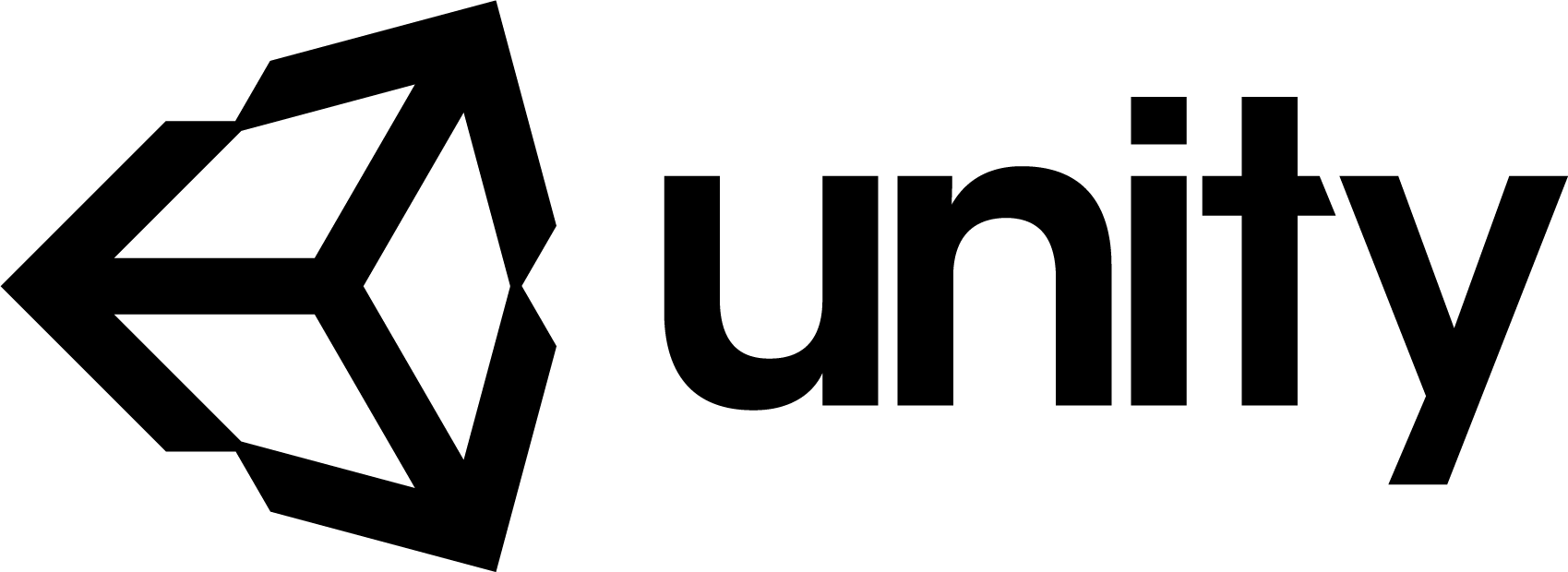## Motivation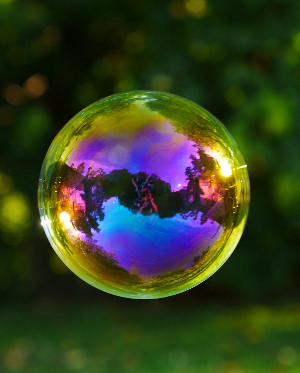Ⓒ zacktionman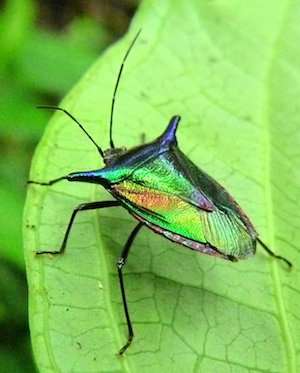Ⓒ Andreas Kay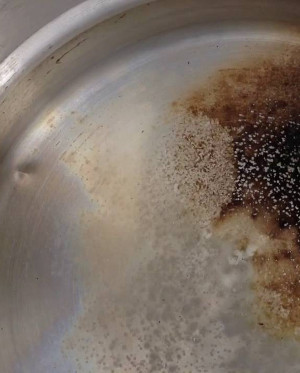Ⓒ BigEmannⒸ Columbia Chemical

## Birth of this Research Project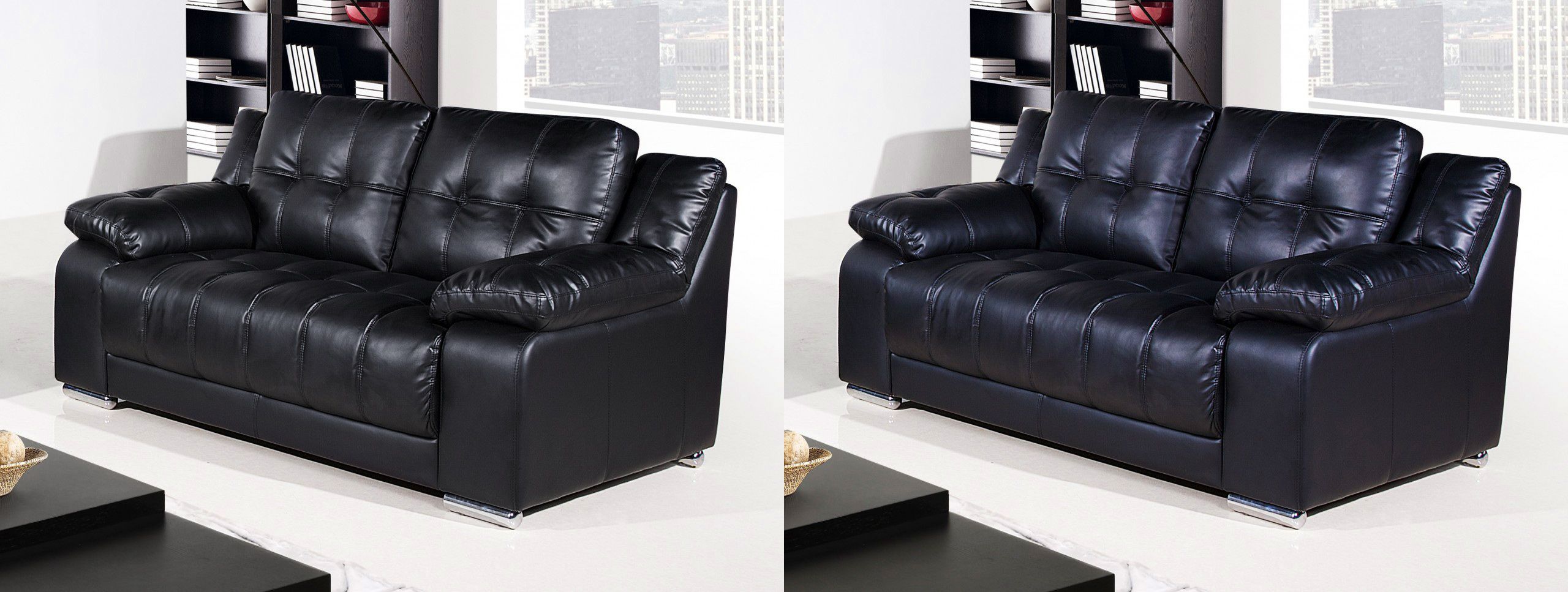Classical microfacets
Iridescent microfacets
• It all started with a blog post
• We wanted to achieve it real-time
• First guess: "should be easy"
• Took us 8 months ...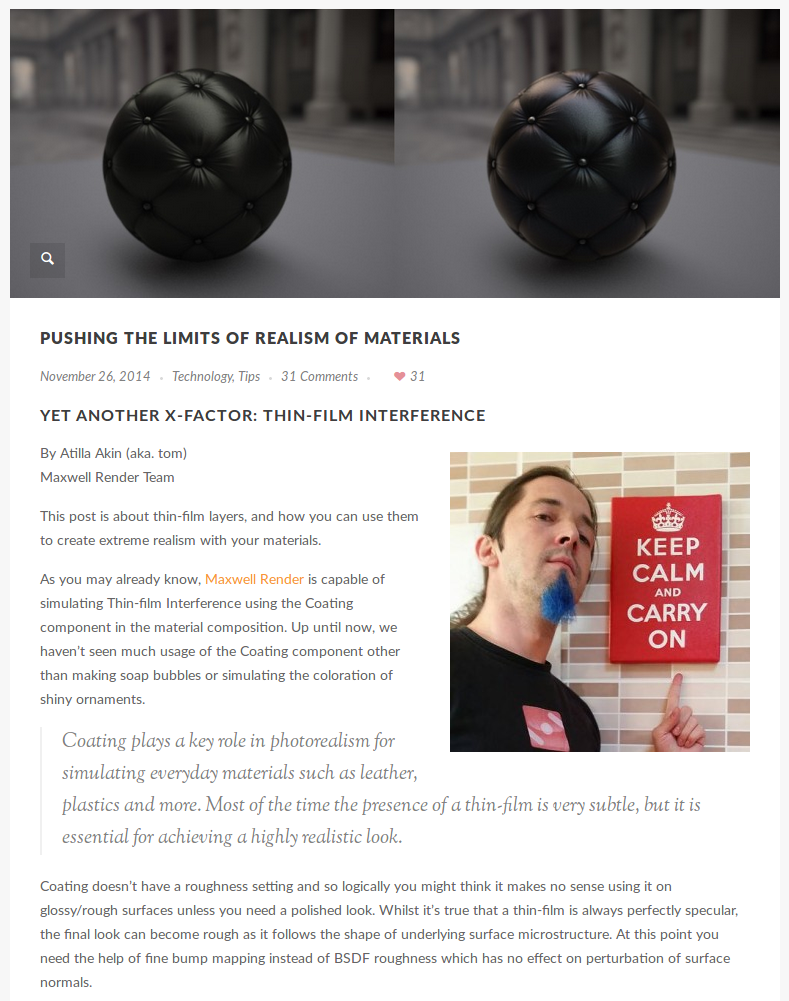Maxwell Render blog

Ⓒ DipYourCar

## Thin-Film Reflectance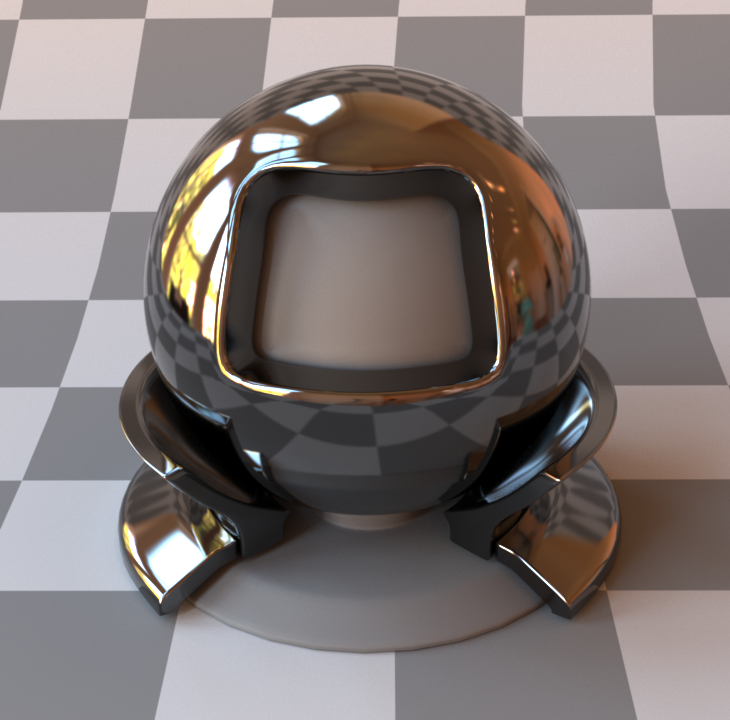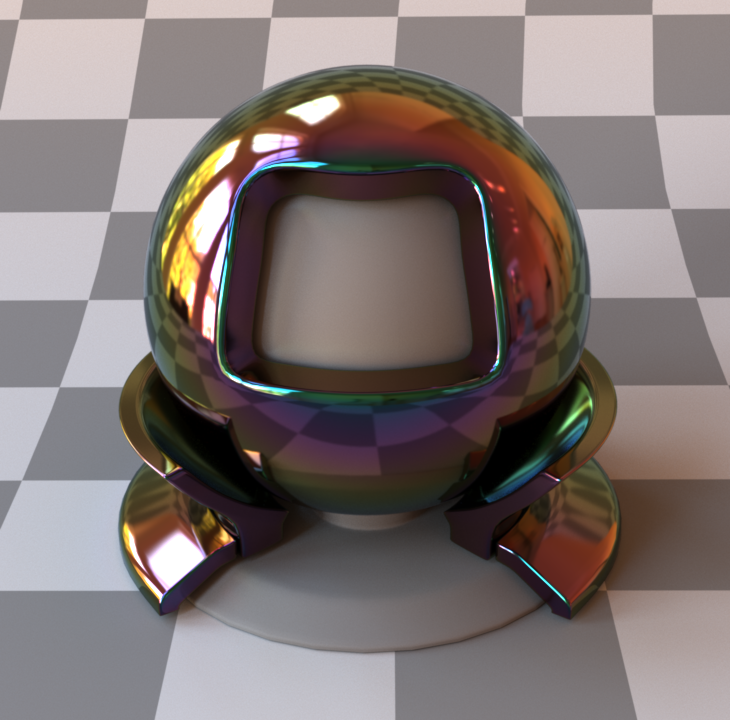## Thin-Film Interference: Using Phase

$\eta_{1}$
$\eta_{2}$
$\vec{\eta}_{3}$
$\mathcal{D}$
$\mathcal{D}$
$\mathcal{D}$
$\mathcal{D}$ is called the Optical Path Difference

## Thin-Film Interference : Airy Summation

$\eta_{1}$
$\eta_{2}$
$\vec{\eta}_{3}$
outgoing reflectance $R = |\vec{r}|^2$

with $\vec{r} = \color{red}{\vec{r}_{12}} + \color{blue}{t_{12}} \color{green}{\vec{r}_{23}e^{i \Delta\phi}} \color{orange}{t_{21}} + \cdots$
$$\vec{r} = \color{red}{\vec{r}_{12}} + \color{blue}{t_{12}} \color{green}{\dfrac{\vec{r}_{23}e^{i \Delta\phi}}{1 - \vec{r}_{21}\vec{r}_{23}e^{i \Delta\phi}}} \color{orange}{t_{21}}$$
Analytical form: Airy summation

## Naive Implementation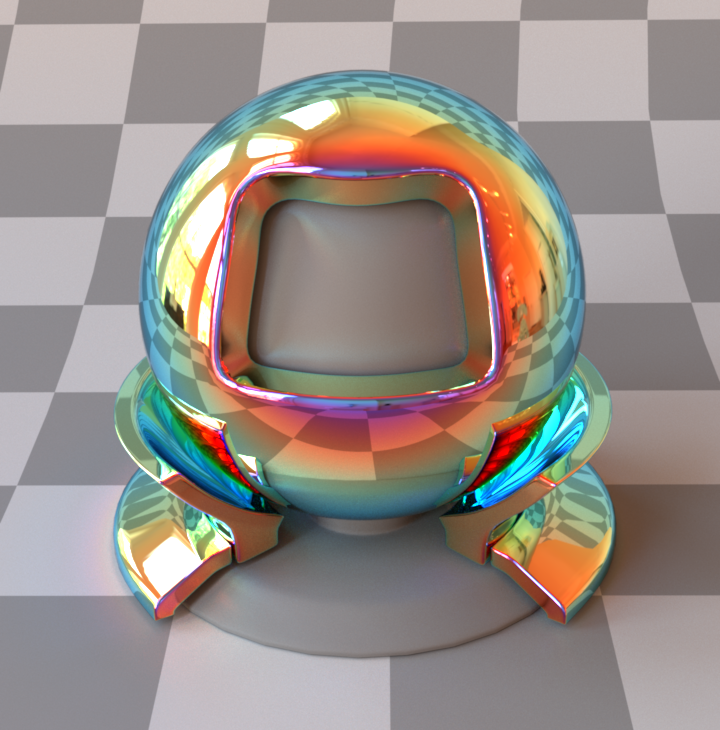RGB renderer - Naive implementation

## Comparison with Spectral Renderer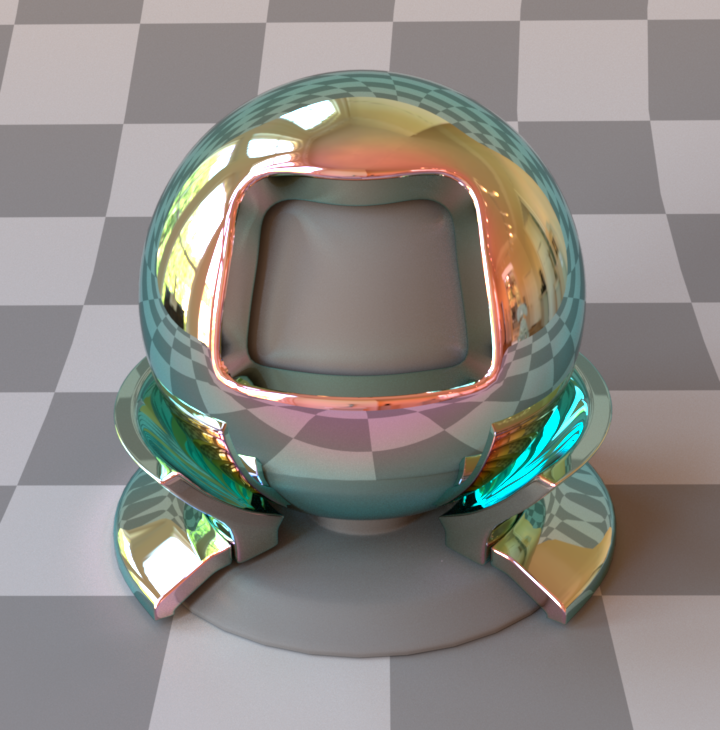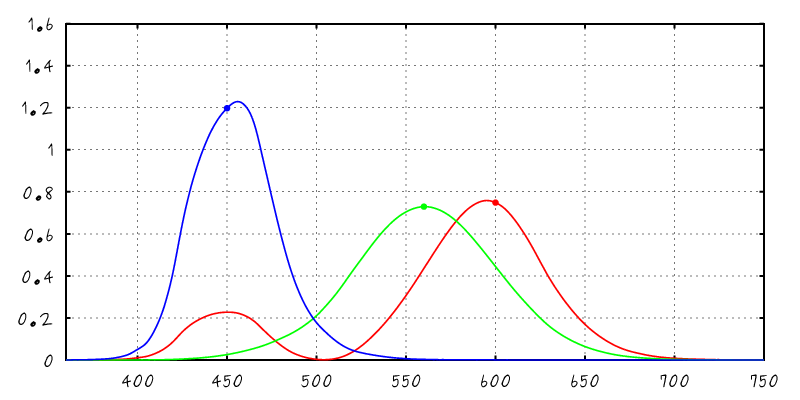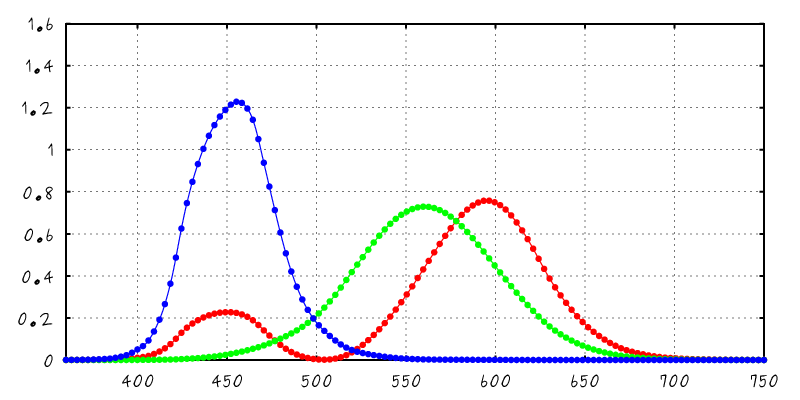RGB renderer - Naive implementation 128 spectral samples - Naive implementation

## Issue: Spectral Aliasing

• Using Naive RGB rendering does spectral aliasing
• Affect goniochromatic materials
• Can be solved using spectral rendering
• Spectral rendering is not an option for video-games
• Our solution: spectral antialiasing
• Account for spectral integration inside the model
• Required a novel view on Airy's summation

## Spectral Integration with Sensor Sensitivity

$$\int$$
$$\times$$
$$\times$$
$$\mbox{d}\lambda$$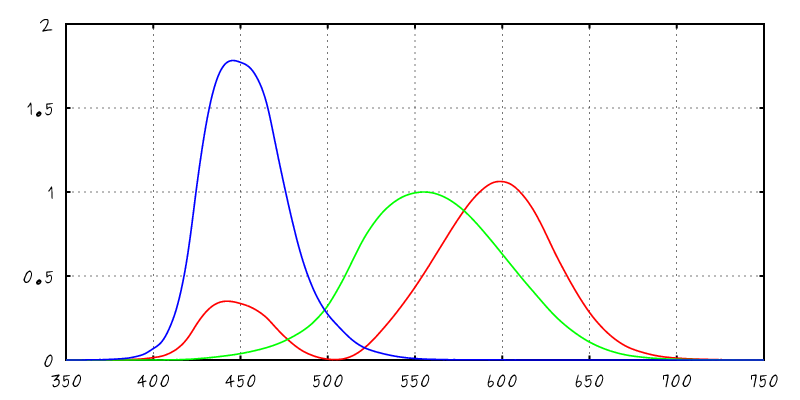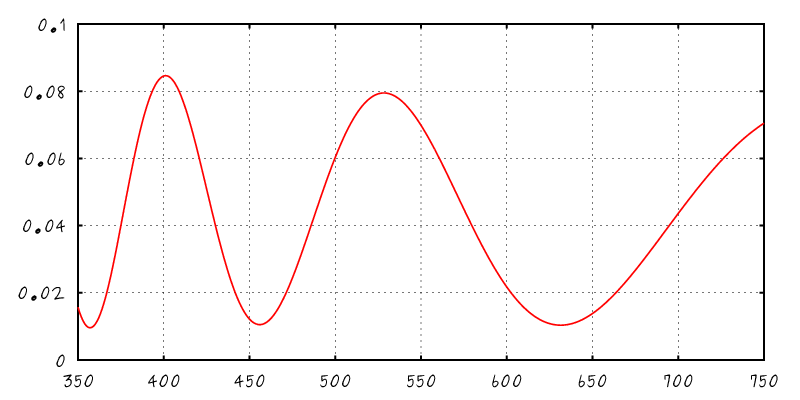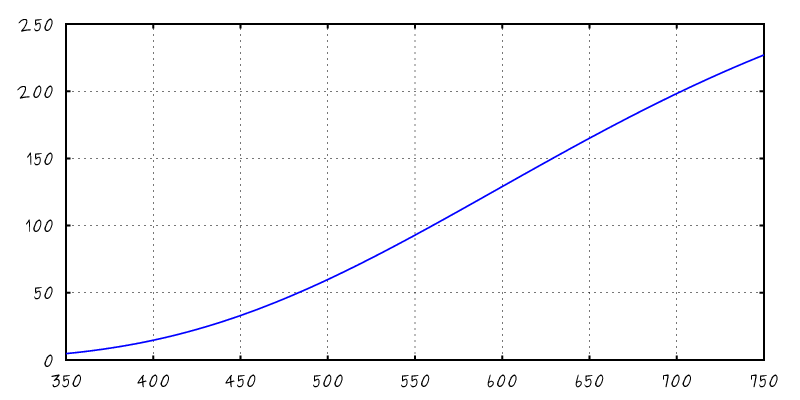XYZ Color Matching Curves Spectral BRDF Illuminant A

## Our Solution: Pre-Integration

• Under approximations
• Illuminant constant per sensitivity function
• Material properties (IORs) constant as well
• We can approximate the spectral integral in closed-form
$$R \! = \! \int \hspace{500px} \mbox{d}\lambda$$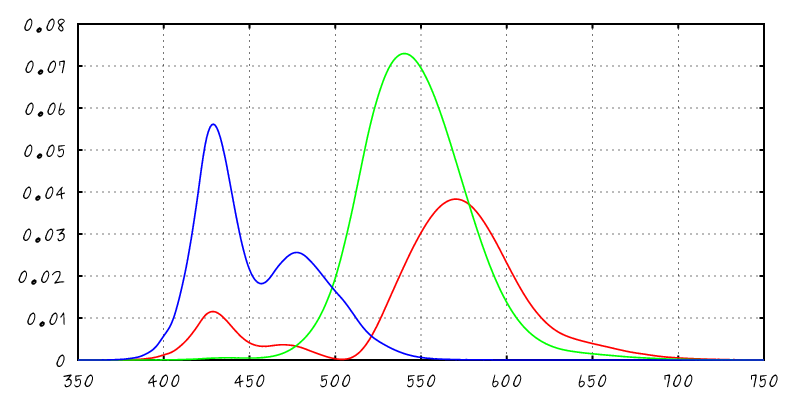XYZ times Reflectance

## Airy Summation Reordered

• Start from Airy summation

$$\vec{r} = \color{red}{\vec{r}_{12}} + \color{blue}{t_{12}} \color{green}{\vec{r}_{23}e^{i \Delta\phi}} \color{orange}{t_{21}} + \cdots$$
• Work on reflectivity and expand summation

$$R = |\vec{r}|^2 = C_0 + \sum_{m = 1}^{+\infty} C_m \color{blue}{\underbrace{\color{black}{\cos(m \Phi)}}}$$
equal phase difference

## Change of Variable

• Express $R$ with respect to light frequency and not wavelength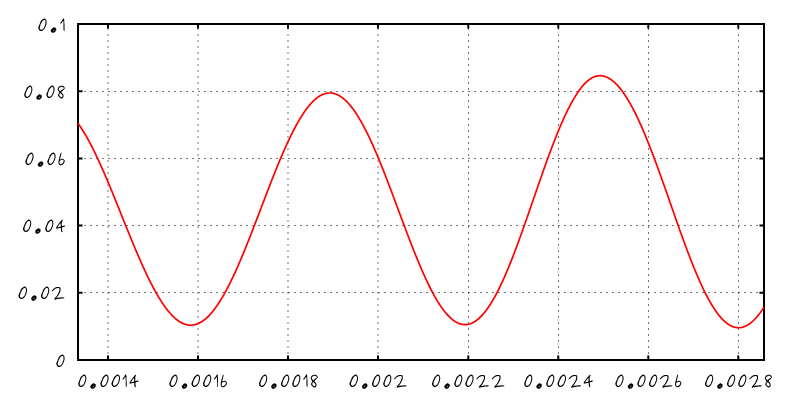$R(\lambda)$ $R(\mu)$ with $\mu \sim \frac{1}{\lambda}$

## Fourier Transform of the Reflectance

• Has discrete form with respect to light frequency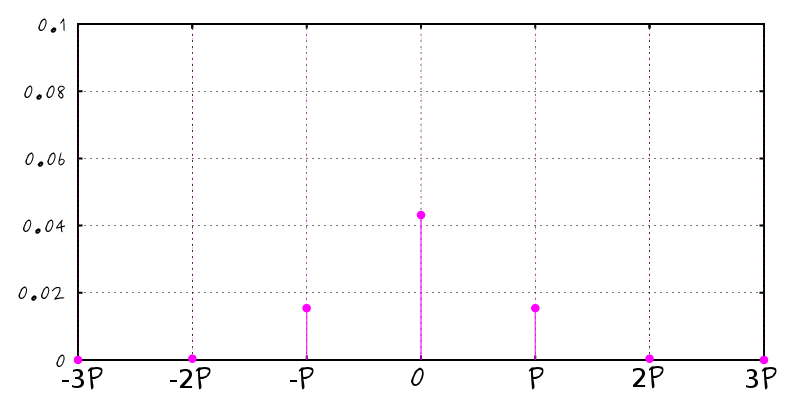$R(\mu)$ with $\mu \sim \frac{1}{\lambda}$ $\mathcal{F}\left[R\right]$

## Integration in Fourier's Space

• Using Parseval's theorem

$$R_j = \int \hat{R}(\mu) \, \overline{\hat{S}_j(\mu)} \, \mbox{d}\mu$$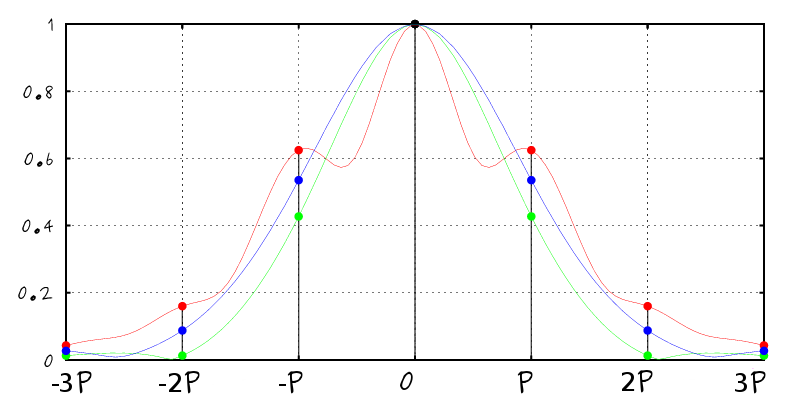Continuous integral Discrete sum
$$\int$$
$$=$$
$$\sum$$

## Integration in Fourier's Space

• Evaluation of the sensitivity's Fourier Transform
• Can be precomputed as a RGB texture
• Can be approximated using 4 Gaussians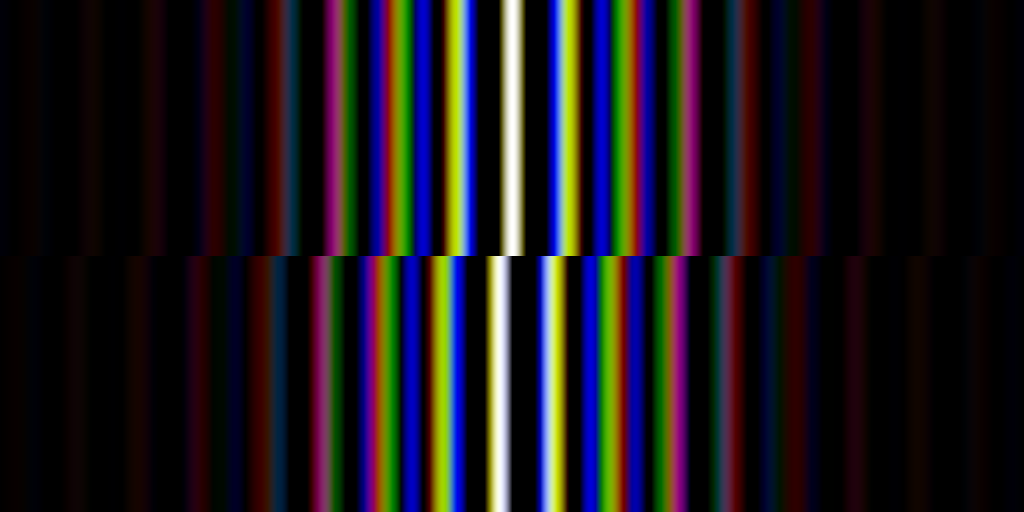Real and imaginary table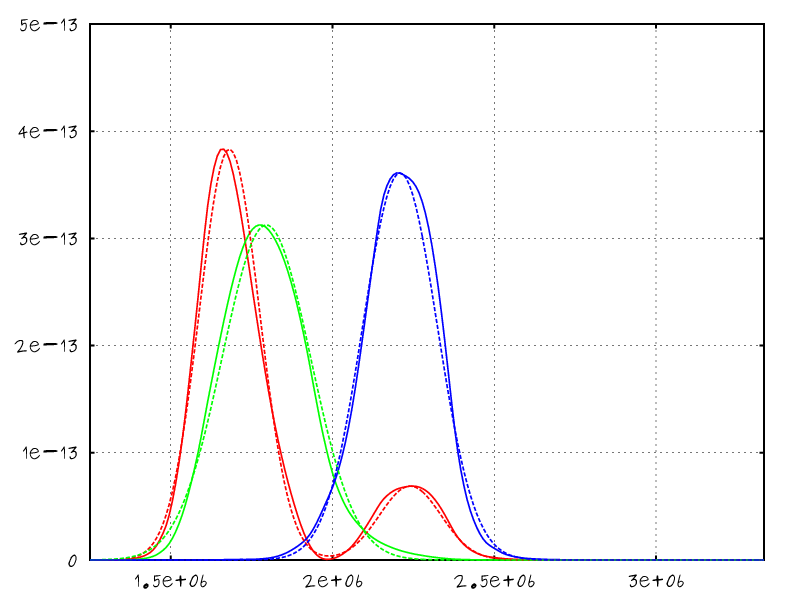Fitting sensitivity functions with Gaussians

## Integration in Microfacet Models

$$\rho = \frac{D(\mathbf{h}) \; G(\pmb{\omega}_i, \pmb{\omega}_o) \; F(\mathbf{h} \cdot \pmb{\omega}_i)}{4 \; (\pmb{\omega}_i \cdot \mathbf{n}) \; (\pmb{\omega}_o \cdot \mathbf{n})}$$
$$\rho = \frac{D(\mathbf{h}) \; G(\pmb{\omega}_i, \pmb{\omega}_o) \color{red}{R_i(\mathbf{h} \cdot \pmb{\omega}_i)}}{4 \; (\pmb{\omega}_i \cdot \mathbf{n}) \; (\pmb{\omega}_o \cdot \mathbf{n})}$$

## Real-Time Rendering Constraints

• Approximation for IBL and Area-Lights
• Decorrelate $R_i$ from the IBL/AL pre-integration
• Evaluate $R_i$ using the mirror direction
• Oversaturate colors for rough materials

## Results: Matlab Validation

• Dielectric film over a dielectric base
• Truncating the infinite sum
• Using up to 3 terms in the series
• In practice: still good up to 2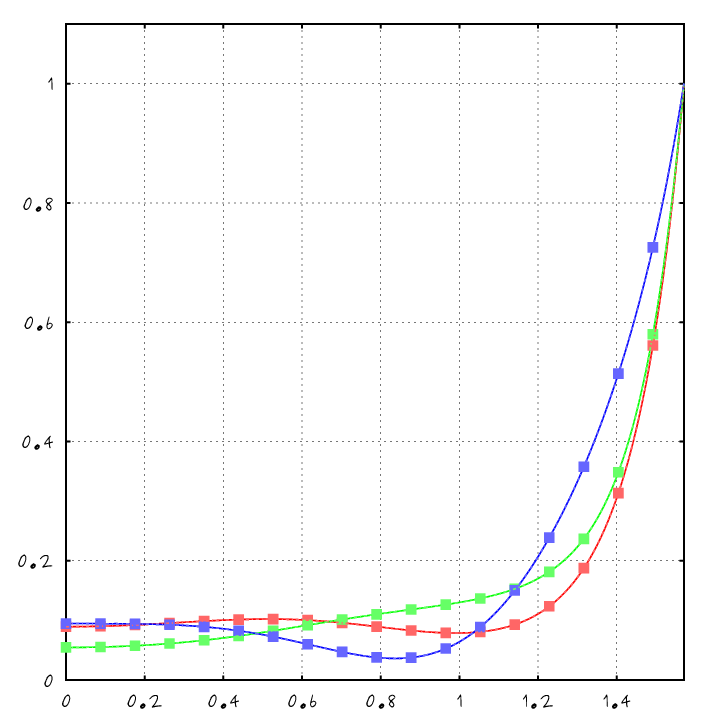XYZ Reflectance w/r to Elevation

## Results: Matlab Validation

• Dielectric film over a dielectric base
• Truncating the infinite sum
• Using up to 3 Optical Path Difference ($\mathcal{D}$)
• In practice: still good up to 2 $\mathcal{D}$
• Also visible in Chromaticity Space
• Display curves w/r $[x,y] = \left[\frac{X}{X+Y+Z}, \frac{Y}{X+Y+Z}\right]$
• Our model faithfully reproduce the GT
• Comparison with Naive RGB
• Fails to correctly reproduce color
• Often goes out of gamut

Chromaticity space x/y

## Results: Offline Validation

• Rendering in Mitsuba
• Conductor base with $\eta = 1.9$ and $\kappa = 1.5$
• Film of thickness $h = 550 \mbox{nm}$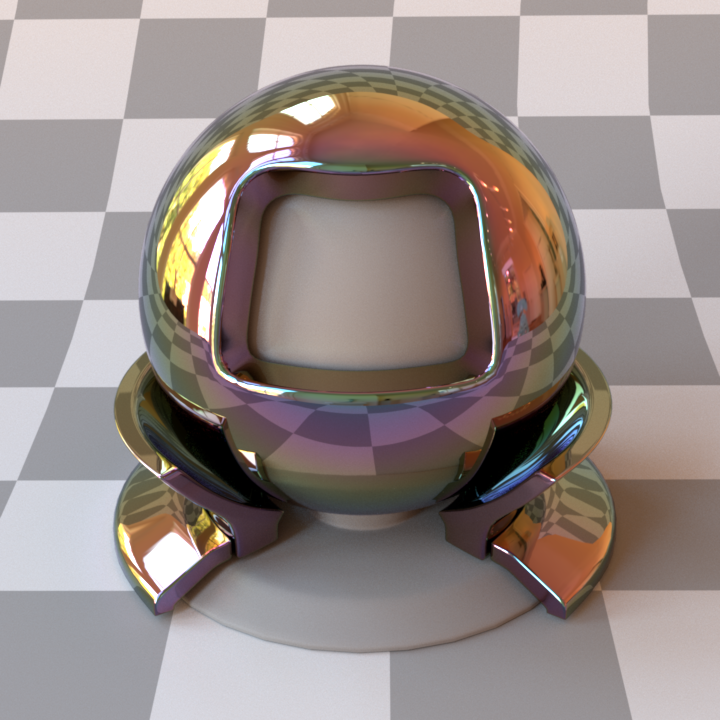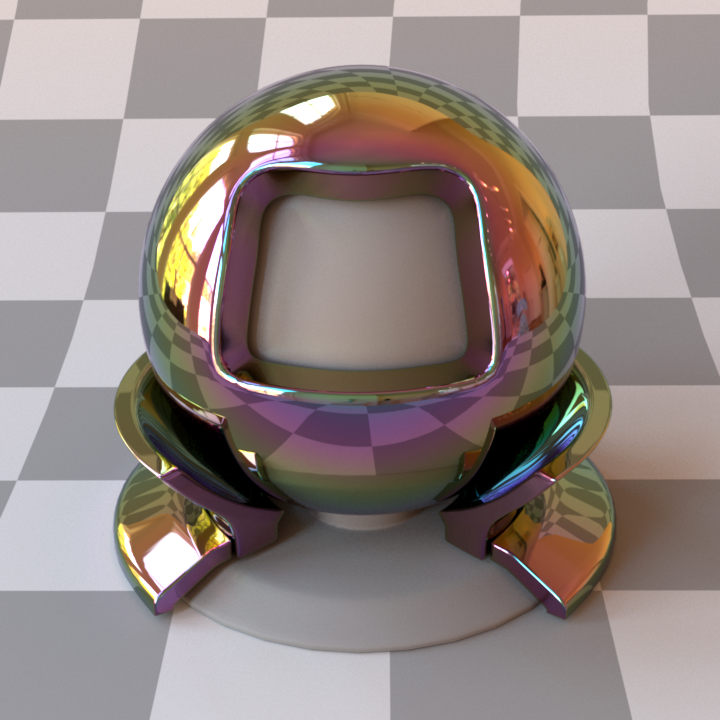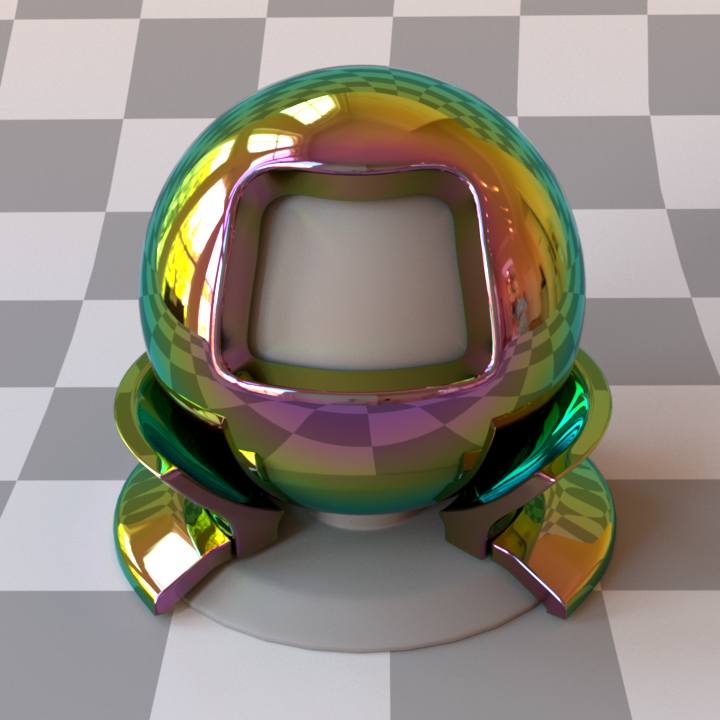Ours (RGB) Ref. (Spectral) [Smits and Meyer 1992]

## Results: Real-Time Validation

• Rendering in BRDF Explorer, Gratin and Unity
• GLSL implementation provided in supp. mat.
• Using the Gaussian approximation of XYZ
• Spatial Variations
• All parameters can be mapped to textures
• In practice: better vary only the thickness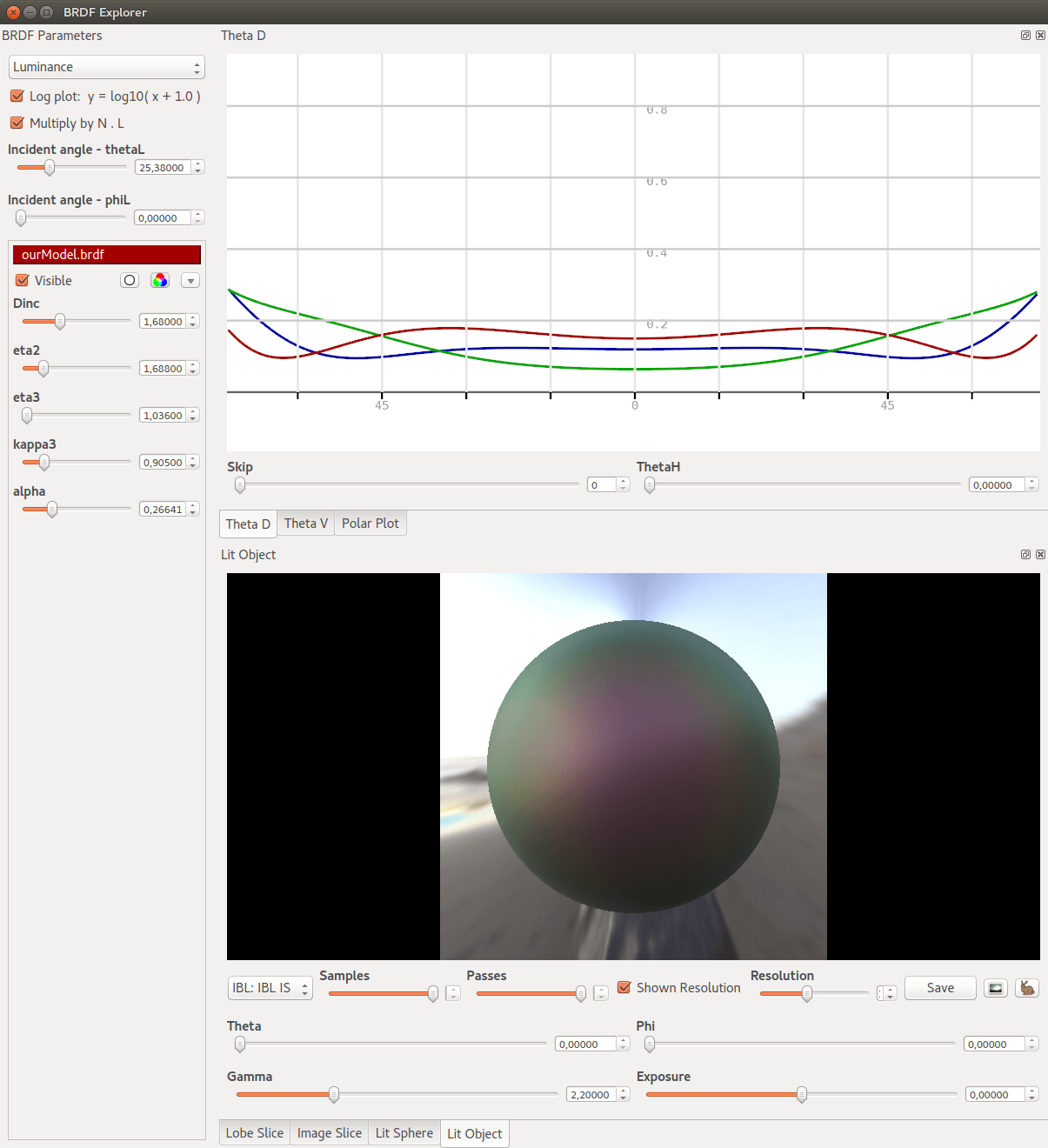## Results: Chair

• Following Maxwell Render team's blog post
• Start from a classical microfacet model and add iridescence
• Similar appearance with same inputs
• Produce a subtle but convincing effect
• Rendered in Mitsuba

## Results: Robot Bust

• Illustrate some possible appearances
• Add texture to modulate thickness
• Rendered in Mitsuba

## Limitations

• Varying Index of Refraction (IOR)
• Using measured data
• Fail to correctly replicated color for highly varying IORs
Glass base Mercury base Copper base

## Summary

• A extension to microfacet models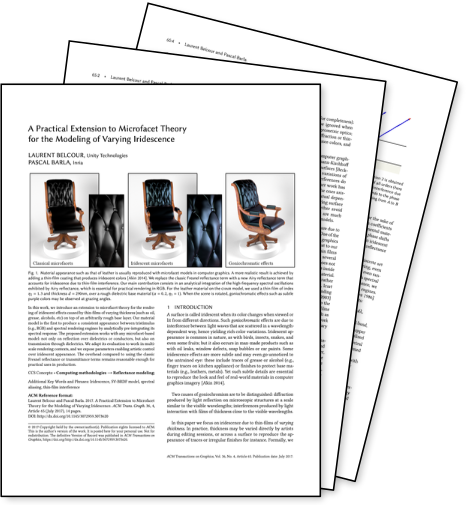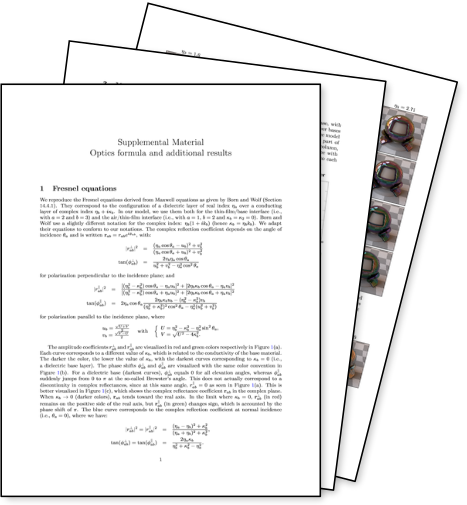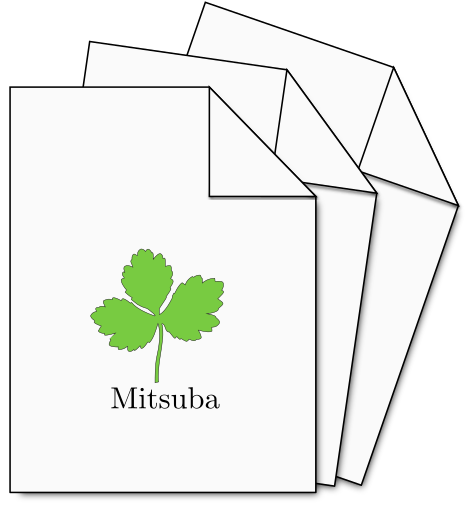paper supp. mat. code